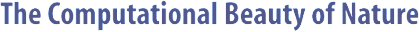Computer Explorations of Fractals, Chaos,Complex Systems, and Adaptation· title page · home* · cover artwork · jacket text · table of contents · the author* · ordering informationBook Contents
 · three themes · part synopses · selected excerpts · all figures from book · quotes from book · glossary from book · bibliography · slide showSource Code
 · overview &documentation · FAQ list* · download source code · java appletsMiscellany
 · news* · reviews & awards · errata · for educators · bibliography (BibTeX format) · other linksPart Synopses

 Computation
 Any discrete piece of information can be represented by a set of numbers. Systems that compute can represent powerful mappings from one set of numbers to another. Moreover, any program on any computer is equivalent to a number mapping. These mappings can be thought of as statements about the properties of numbers; hence, there is a close connection between computer programs and mathematical proofs. But there are more possible mappings than possible programs; thus, there are some things that simply cannot be computed. The actual process of computing can be defined in terms of a very small number of primitive operations, with recursion and/or iteration comprising the most fundamental pieces of a computing device. Computing devices can also make statements about other computing devices. This leads to a fundamental paradox that ultimately exposes the limitations not just of of machine logic, but all of nature as well. Chapter 2 introduces some important properties of different types of numbers, sets, and infinities. Chapter 3 expands on this by introducing the concepts behind computation and shows how computation can be seen to operate over sets of integers. Chapter 4 ties together some of the paradoxes seen in the earlier chapters to show how they are applicable to all of mathematics.

 Fractals
 Euclidean geometry can be extended to account for objects with a fractional dimension. Such objects, known as fractals, come very close to capturing the richness and variety of forms found in nature. Fractals possess structural self-similarity on multiple spatial scales, meaning that a piece of a fractal will often look like the whole. Related to this property is the fact that fractals are extremely compressible in the sense that an algorithm or recipe for the image is far simpler to store than the image itself. This is due to the recursive and/or iterative nature of the fractal algorithm. But since fractals are so closely tied to algorithms, some fractals have the same paradoxical properties as systems that can compute. Chapter 5 introduces some of the basic ideas behind fractal geometry by considering some unusual geometric objects as well as some common stochastic processes found in nature. Chapter 6 focuses on L-systems, which are simple program fragments that can define plantlike and other fractals. Chapter 7 considers fractals that can be defined by linear algebra operators, and Chapter 8 focuses on two special types of nonlinear fractals. This part is concluded by 9, which examines how fractals can be seen as ``nearly simple'' objects by some candidate measures of complexity.

 Chaos
 Simple motion is rare in nature. It is more common to find highly complicated nonlinear motion due to chaos. Chaotic systems can easily be mistaken for randomness despite the fact that they are always deterministic. Part of the confusion is due to the fact that the future of chaotic systems can be predicted only on very short-term time scales. Chaotic systems possess a form of functional self-similarity that shows itself in fractal strange attractors. This fractal functionality, combined with chaotic unpredictability, is reminiscent of the uncertainty found in computing systems. Chapter 10 introduces the key components of chaotic systems and highlights the properties common to all such systems. Chapter 11 contains a sampling of fractal strange attractors that are generated from chaotic systems. Chapter 12 considers a type of chaos found in producer-consumer systems, and compares and contrasts two very different types of modeling methods. Chapter 13 shows how some of the properties of chaotic systems can be exploited for the purpose of controlling them. Finally, Chapter 14 compares and contrasts chaos to randomness and incomputability.

 Complex Systems
 Complex systems are things that consist of many similar and simple parts. Often the underlying behavior of any of the parts is easily understood, while the behavior of the system as a whole defies simple explanation. Part of the reason why complex systems can behave in such complicated ways is that some general forms are known to be capable of universal computation. This double-edged result also implies that the future of complex systems cannot be predicted in the general case. By changing the type and form of interactions that exist among the parts of a complex system, the type of global behavior can be varied such that the complex system as a whole can be globally goal-seeking while only local information is passed around by the parts. This means that a collective form of computation can take place without an explicit global algorithm. Chapter 15 introduces a simple and nearly canonical form of a complex system, known as a cellular automaton. Chapter 16 shows how collective systems composed of individual agents can demonstrate self-organized behavior. Chapter 17 reveals how mixtures of competition and cooperation can produce many different and interesting types of behavior that are seen in nature. Chapter 18 illustrates how a collective form of computation can be performed by feedback neural networks with fixed synapses. Finally, Chapter 19 examines how the global form and function of composite objects can be influenced by the parts of the system and the types of interactions permitted.Copyright © Gary William Flake, 1998-2002. All Rights Reserved.Last modified: 30 Nov 2002